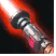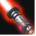# [gamemaster]Conditions

## Related effects, buffs and debuffs

• [900s][gamemaster]

 Slot: Buff Duration: 15m 0s Tick rate: 60s Initial stacks: 15 Max stacks: 15 # occurrences: 0 Target overrides: [target override] Aoe Sphere
• When effect is applied
• [target override]

Only when the following conditions are met:

• <
• If target is an enemy
AND
• If PRIMARY TARGET is a player
>

Perform the following actions:

• Execute Hydra Hook
- Event Name = (string) EmpireSpawn
- Object Self = (int) 2
• Custom
- Param 4 = (string) Debug: Teleport Empire
- Param 5 = (string)
- Param 6 = (string)
- Param 7 = (string)
- Param 8 = (string)
- Param 9 = (string)
- Param 1 = (int) 10199437207600779921
- Param 2 = (int) 7044208247444806032
- Param 3 = (int) 1078249248256508798
• When effect is applied
• [target override]

Only when the following conditions are met:

• <
• If PRIMARY TARGET is a player
AND
• If TARGET is a friend of CASTER
>

Perform the following actions:

• Execute Hydra Hook
- Event Name = (string) RepublicSpawn
- Object Self = (int) 2
• Custom
- Param 4 = (string) Debug: Teleport Republic
- Param 5 = (string)
- Param 6 = (string)
- Param 7 = (string)
- Param 8 = (string)
- Param 9 = (string)
- Param 1 = (int) 10199437207600779921
- Param 2 = (int) 7044208247444806032
- Param 3 = (int) 1078249248256508798
• When effect is applied

Perform the following actions:

• Custom
- Param 4 = (string) The Warzone Match Has Begun!
- Param 5 = (string)
- Param 6 = (string)
- Param 7 = (string)
- Param 8 = (string)
- Param 9 = (string)
- Param 1 = (int) 10199437207600779921
- Param 2 = (int) 7044208247444806032
- Param 3 = (int) 1078249248256508798
• Grant Immunity
- Tag = (string)
- Ability Spec = (int) 0
- Damage Type List = Int(5)
- Damage Channel List = Int(1)
• Immobilize but allow rotation
• Put TARGET into stealth
• When effect ticks

Perform the following actions:

• Modify Charges
- Tag = (string)
- Ability Spec = (int) 0
- Amount Max = (float) -1
- Amount Min = (float) -1
- Amount Percent = (float) 0
• When stacks of this effect decrease
• When effect is applied

Perform the following actions:

• Custom
- Param 4 = (string) minute(s) remaining.
- Param 5 = (string)
- Param 6 = (string)
- Param 7 = (string)
- Param 8 = (string)
- Param 9 = (string)
- Param 1 = (int) 10199437207600779921
- Param 2 = (int) 7044208247444806032
- Param 3 = (int) 7777366219083085155
• When effect expires

Perform the following actions:

• Execute Hydra Hook
- Event Name = (string) TimerExpires
- Object Self = (int) 2
• Custom
- Param 4 = (string) The Timer Has Expired!
- Param 5 = (string)
- Param 6 = (string)
- Param 7 = (string)
- Param 8 = (string)
- Param 9 = (string)
- Param 1 = (int) 10199437207600779921
- Param 2 = (int) 7044208247444806032
- Param 3 = (int) 1078249248256508798
• Custom
- Param 4 = (string) Warzone Match Results:
- Param 5 = (string)
- Param 6 = (string)
- Param 7 = (string)
- Param 8 = (string)
- Param 9 = (string)
- Param 1 = (int) 10199437207600779921
- Param 2 = (int) 7044208247444806032
- Param 3 = (int) 1078249248256508798
• When effect expires
• [target override]

Only when the following conditions are met:

• If PRIMARY TARGET is not a player

Perform the following actions:

• Instantly kill the TARGET